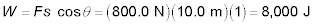##### Physics I Workbook For Dummies with Online Practice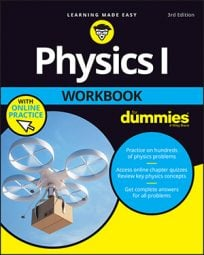In physics, physical work is defined as the applied force multiplied by the component of the displacement that is in the same direction as the force. So if you’re pushing a refrigerator 2.0 m across the floor, and you need to apply 900 N, you’ve done this much work:

(900)(2.0) = 1,800

1,800 what? Work in the MKS system is measured in joules, J, so that’s 1,800 J. You will almost always see work expressed in joules in physics problems. The CGS unit for work is the (oddly named) erg, but it’s rare that you’ll see that used. (The FPS unit for work is the foot-pound.)

So applying a force of 1.0 N over a distance of 1.0 m means that you’ve done 1 joule of work. 1.0 J = 1.0 x 107 ergs because ergs are dyne-centimeters, and there are 105 dynes per Newton and 102 centimeters in a meter.

But work isn’t just the total force applied multiplied by the distance traveled; the only component of a force that counts is the one applied along the direction of travel. For example, look at the mass being pulled in the following figure.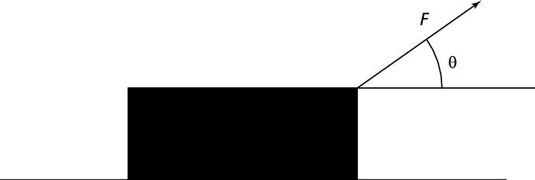The force applied along the direction of travel is what counts, so you want the component of F that is horizontal (assuming you’re dragging the mass and not picking it up!). That horizontal component is F cos theta, so the work you do, W, is this: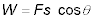Here, F is the force applied, s is the displacement, and theta is the angle between them.

## Sample question

1. Suppose that you’re pulling the mass in the figure by applying 200. N and the angle theta is 40.0 degrees. How much work do you do dragging the mass over 50.0 m?

The correct answer is 7,660 J.

1. Use the equation2. Plug in the numbers: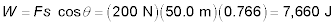## Practice questions

1. You’re pulling a chest of drawers, applying a force of 60.0 N at an angle of 60.0 degrees. How much work do you do pulling it over 10.0 m?

2. You’re dragging a sled on a rope at 30 degrees, applying a force of 20 N. How much work do you do over 1.0 km?

3. 3.You’re pushing a box of dishes across the kitchen floor, using 100.0 J to move it 10.0 m. If you apply the force at 60.0 degrees, what is the force you used?

4. You’re pushing an out-of-gas car down the road, applying a force of 800.0 N. How much work have you done in moving the car 10.0 m?

Following are answers to the practice questions:

1. 300 J

1. Use the equation2. Plug in the numbers: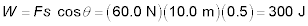2. 1.7 x 104 J

1. Use the equation2. Plug in the numbers: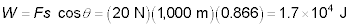3. 20 N

1. Use the equation2. Solve for force: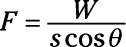3. Plug in the numbers: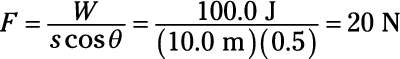4. 8,000 J

1. Use the equation2. Plug in the numbers: